## Python 机器学习实战 —— 无监督学习（上）

1.1 PCA 降维的基本原理

PCA 会根据设置把数据点分解成一些分量的加权求和，利用降维的思想，把多指标转化为少数几个综合指标。这个变换把数据变换到一个新的坐标系统中，使得任何数据投影的第一大方差在第一个坐标(称为第一主成分)上，第二大方差在第二个坐标(第二主成分)上，依次类推。主成分分析经常用于减少数据集的维数，同时保持数据集的对方差贡献最大的特征。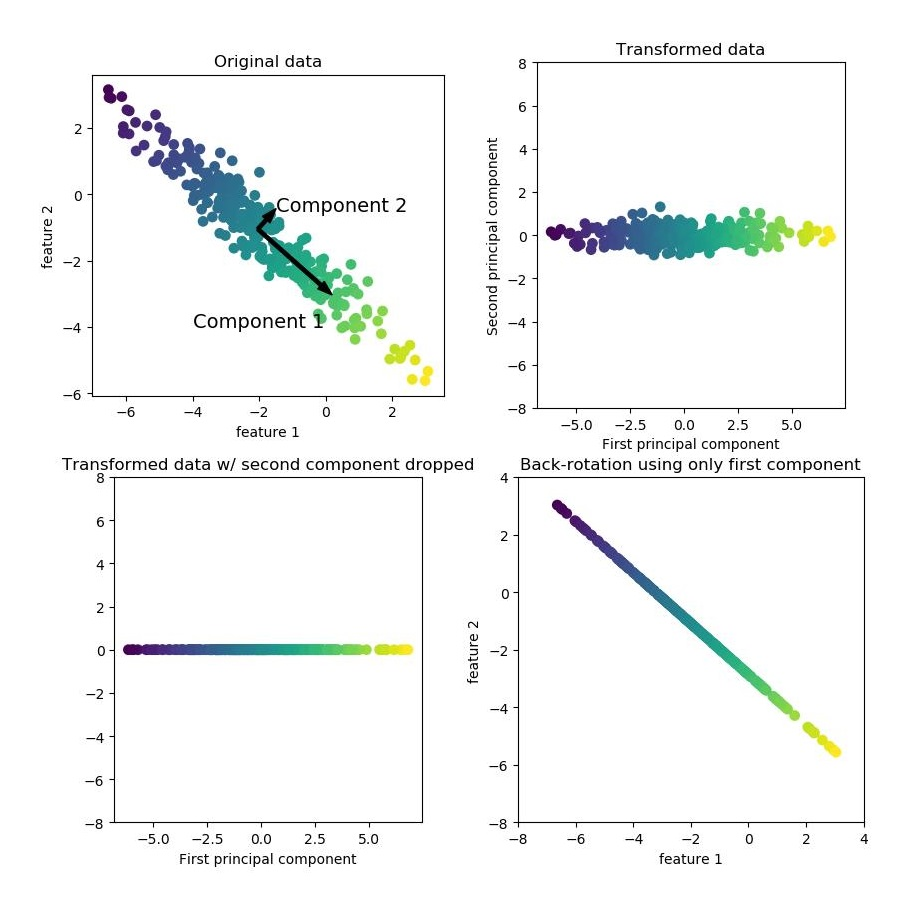1.2 PCA 类简介

1 class PCA(_BasePCA):
2     @_deprecate_positional_args
3     def __init__(self, n_components=None, *, copy=True, whiten=False,
4                  svd_solver='auto', tol=0.0, iterated_power='auto',
5                  random_state=None):
6     ......

• n_components：int, float 类型 or 'mle', 默认值国 None。int 时则是直接指定希望PCA降维后的特征维度数目，此时 n_components是一个大于等于1的整数。也可以使用 float 指定主成分的方差和所占的最小比例阈值，让PCA类自己去根据样本特征方差来决定降维到的维度数，此时 n_components是一个[0，1]之间的数。当然，我们还可以将参数设置为"mle", 此时PCA类会用MLE算法根据特征的方差分布情况自己去选择一定数量的主成分特征来降维。我们也可以用默认值，即不输入n_components，此时n_components=min(样本数，特征数)。
• copy: bool 类型，表示是否在运行算法时，将原始数据复制一份。默认为True，则运行PCA算法后，原始数据的值不会有任何改变。因为是在原始数据的副本上进行运算的。
• whiten ：bool 类型，判断是否进行白化，默认值为 False ，即不进行白化。所谓白化，就是对降维后的数据的每个特征进行归一化，让方差都为1.对于PCA降维本身来说，一般不需要白化。如果你PCA降维后有后续的数据处理动作。
• svd_solver：str 类型，可选值 {'auto', 'full', 'arpack', 'randomized'} 之一，默认值为 auto 。即指定奇异值分解SVD的方法，由于特征分解是奇异值分解SVD的一个特例，一般的PCA库都是基于SVD实现的。randomized一般适用于数据量大，数据维度多同时主成分数目比例又较低的PCA降维，它使用了一些加快SVD的随机算法。 full则是传统意义上的SVD，使用了scipy库对应的实现。arpack和randomized的适用场景类似，区别是randomized使用的是scikit-learn自己的SVD实现，而arpack直接使用了scipy库的sparse SVD实现。默认是auto，即PCA类会自己去在前面讲到的三种算法里面去权衡，选择一个合适的SVD算法来降维。一般来说，使用默认值就够了。
• tol: float 类型，默认值为 0.0，代表求解方法精度。
• iterated_power: int 类型，默认auto，代表当 svd_solver == ‘randomized’ 时幂方法的迭代次数。
• random_state: int 类型，默认None，随机数种子，推荐设置一个任意整数，同一个随机值，模型可以复现。

• components_：返回主要成分，运行 transform 后的主要成分将保存在此属性当中。
• n_components_：返回所保留的成分个数 n。
• explained_variance_：特征方差值，方差值越大，说明重要性要越高。与 explained_variance_ratio_ 同时使用可更清晰分辨每个特征的占比。
• explained_variance_ratio_：每个特征方差贡献率，个比例越大，说明重要性要越高，所有总和为1。
• noise_variance_:  根据概率主成分分析模型估计的噪声协方差。

• fit(self, X, y=None):   表示用数据X来训练PCA模型。
• transform(selft,X)：将数据X转换成降维后的数据。通过与 fit 同用，先调用 fix，当模型训练好后，再使用 transform 方法来降维。
• fit_transform(self, X, y=None):　用X来训练PCA模型，同时返回降维后的数据。相当于结合了 fit 与 transform 两个方法。
• inverse_transform(self, X)：将降维后的数据转换成为原始数据。

1.3 应用实例

1.3.1 使用 PCA 降噪

 1 def knn_test():
2     #测试数据集
3     person=datasets.fetch_lfw_people(min_faces_per_person=20)
4     X_train,X_test,y_train,y_test=train_test_split(person.data,person.target,random_state=1)
5     #使用 KNeighborsClassifier 模型进行测试
6     knn = KNeighborsClassifier()
7     knn.fit(X_train, y_train)
8     #输出knn准确率
9     print('KNN:\n  train data:{0}\n  test data:{1}'
10           .format(knn.score(X_train, y_train),
11                   knn.score(X_test, y_test)))
12
13 def pca_test():
14     #测试数据集
15     person=datasets.fetch_lfw_people(min_faces_per_person=20)
16     X_train,X_test,y_train,y_test=train_test_split(person.data,person.target,random_state=1)
17     #建立PCA模型，使用60个主要成分
18     pca=PCA(60 ,whiten=True,random_state=2).fit(X_train)
19     #将数据X转换成降维后的数据
20     X_train_pca=pca.transform(X_train)
21     X_test_pca=pca.transform(X_test)
22     #建立 KNeighborsClassifier 模型
23     knn=KNeighborsClassifier()
24     #把过滤后60个特征的数据放入KNN模型进行训练
25     knn.fit(X_train_pca,y_train)
26     #输出准确率
27     print('\nPCA->KNN:\n  train data:{0}\n  test data:{1}'
28           .format(knn.score(X_train_pca, y_train),
29                   knn.score(X_test_pca, y_test)))
30     #观察累计方差贡献率
31     plt.plot(np.cumsum(pca.explained_variance_ratio_))
32     plt.xlabel('number of components')
33     plt.ylabel('cumulative explained variance')
34     plt.show()
35
36 if __name__ == '__main__':
37     knn_test()
38     pca_test()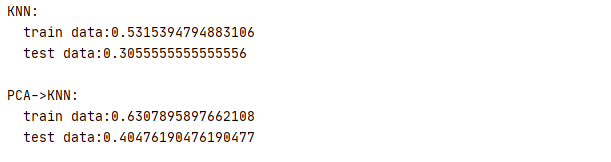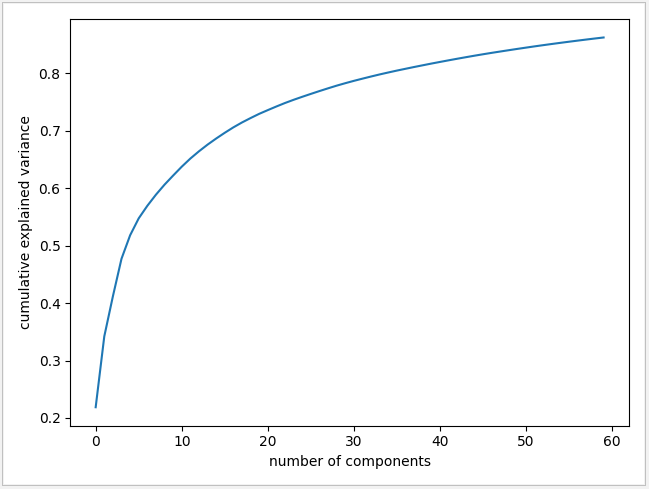1.3.2 提取面部特征

PCA 通过 fit_transform 方法完成降维后，可通过 inverse_transform 方法将降维后的数据转换成为原始数据。对比一下图一与图二，可以辨别二种不同的情况，通过使用此方法对图片进行压缩，原来 3023 个特征已经被压缩到 175 个，图片的特征已比较清晰。特征成分保存在 PCA 的 components_ 属性中，图三显示了前 15 个特征成分。

 1 def pca_test():
2     #测试数据集
3     person=datasets.fetch_lfw_people(min_faces_per_person=20)
4
5     #建立PCA模型，使用 95% 的主要成分
6     pca=PCA(0.95 ,whiten=True,random_state=2)
7     #将数据X转换成降维后的数据
8     componmets=pca.fit_transform(person.data)
9     #显示原图
10     index1,index2,index3=0,0,0
11     fix, axes1 = plt.subplots(3, 5, figsize=(60, 40))
12     for ax in axes1.ravel():
13         a=person.data[index1,:]
14         ax.imshow(person.data[index1,:].reshape(62,47),cmap='viridis')
15         ax.set_title('original '+str(index1),fontsize=100)
16         index1+=1
17     #提取特征成分后还原数据
18     fix, axes2 = plt.subplots(3, 5, figsize=(60, 40))
19     for ax in axes2.ravel():
20         image = pca.inverse_transform(componmets[index2])
21         ax.imshow(image.reshape(62, 47), cmap='viridis')
22         ax.set_title('inverse '+str(index2),fontsize=100)
23         index2 += 1
24     #显示特征成分
25     fix, axes3 = plt.subplots(3, 5, figsize=(60, 40))
26     for ax in axes3.ravel():
27         ax.imshow(pca.components_[index3].reshape(62, 47), cmap='viridis')
28         ax.set_title('component ' + str(index3), fontsize=100)
29         index3 += 1
30     plt.show()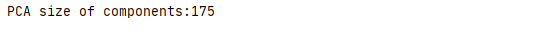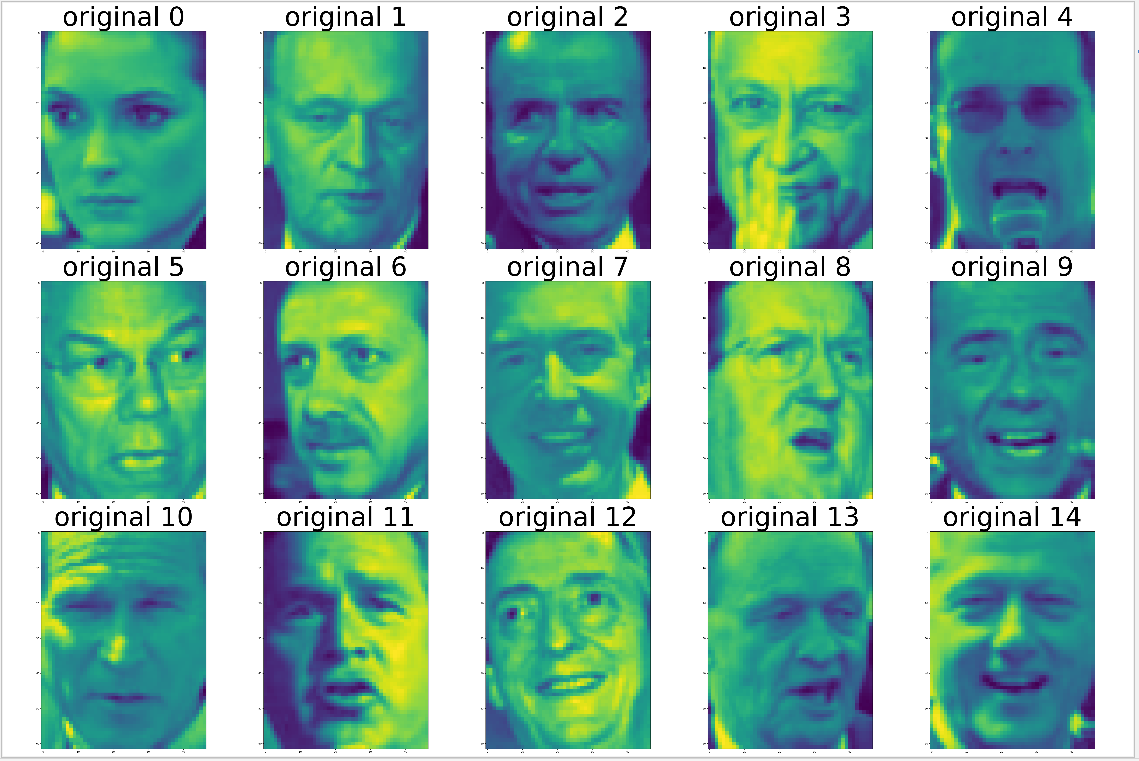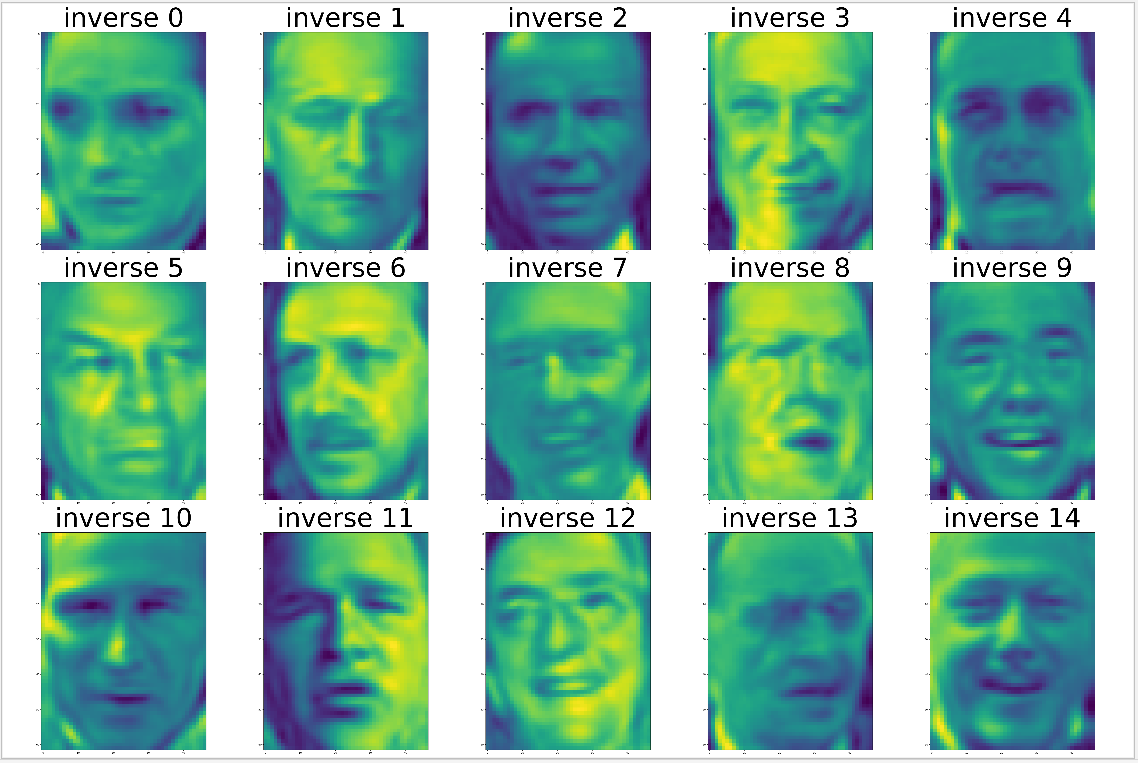特征成分图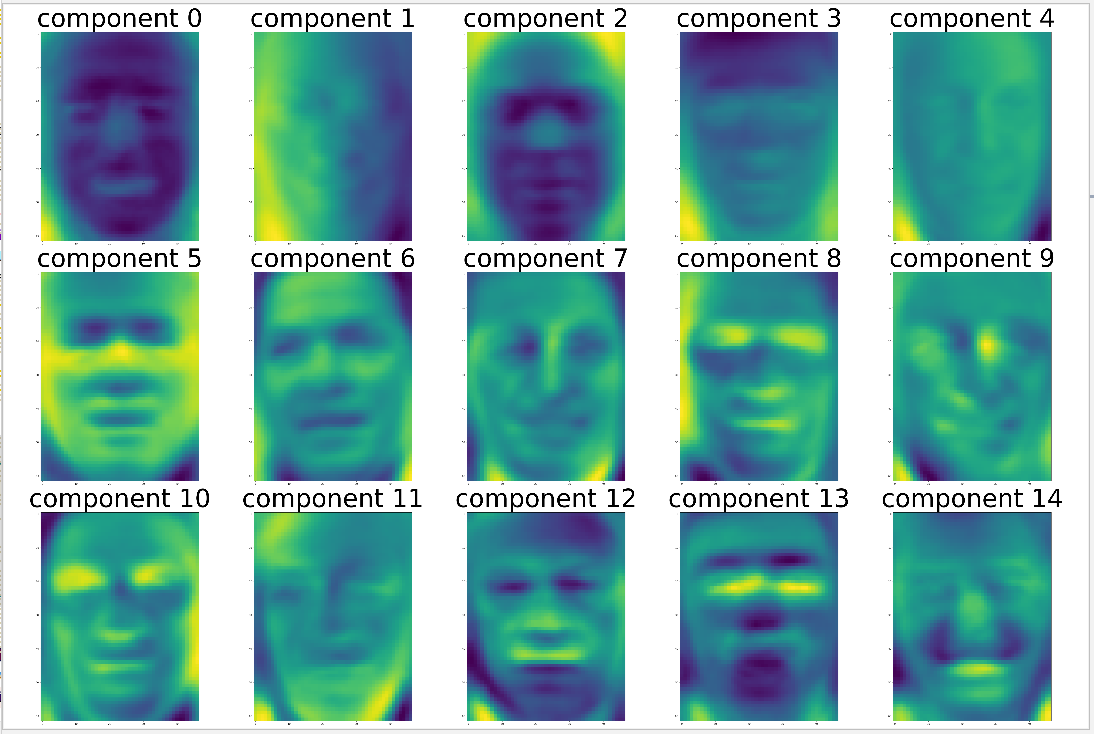2.1 NMF 基本原理

NMF 使用了随机初始化，不同的随机种子可能产生不同的结果。对于两个分量的 NMF 如左图，所有的数据点都可以分解成两个正数组合。特征数越多，分量个数越多，只要有足够多的分量，特征就可以完成地分解。如果仅使用一个分量如右图，NMF 就会创建一个指向平均值的分量。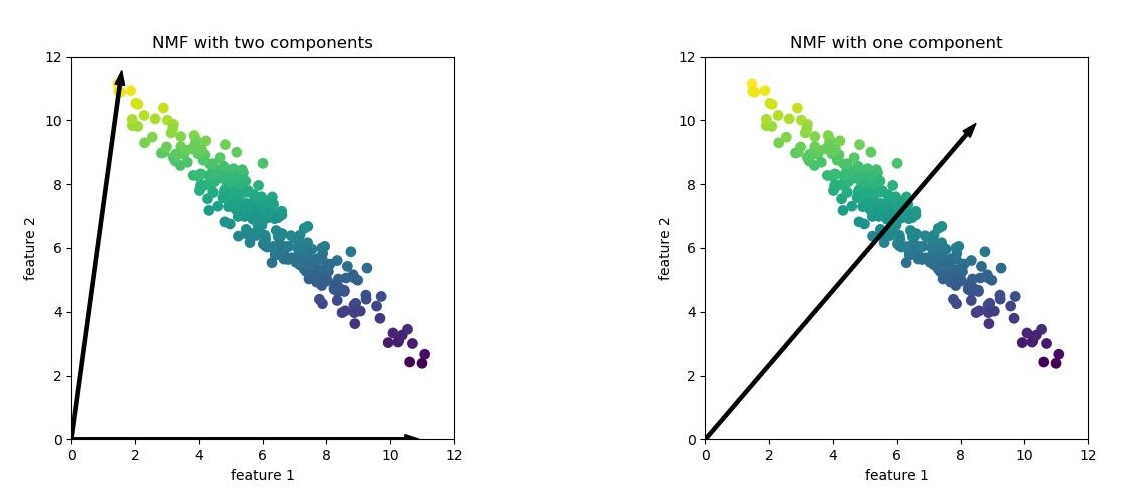2.2 NMF 类简介

1 class NMF(TransformerMixin, BaseEstimator):
2     @_deprecate_positional_args
3     def __init__(self, n_components=None, *, init='warn', solver='cd',
4                  beta_loss='frobenius', tol=1e-4, max_iter=200,
5                  random_state=None, alpha=0., l1_ratio=0., verbose=0,
6                  shuffle=False, regularization='both'):

• n_components：int, 默认值为 None。int 时则是直接指定 NMF 降维后的特征维度数目，此时 n_components是一个大于等于 1 的整数。当使用默认值，即不输入n_components，此时n_components=min(样本数，特征数)。
• init: str 类型，可选择 {'random', 'nndsvd', 'nndsvda', 'nndsvdar', 'custom'} 之一，默认为 None。选择W,H迭代初值的算法， 当为 None 时，即自动选择值，不使用选择初值的算法。'random'：非负随机矩阵，缩放为：sqrt(X.mean() / n_components); ‘nndsvd’：非负双奇异值分解(NNDSVD)，初始化（对于稀疏性更好); 'nndsvda'：NNDSVD与零填充了X的平均值(当不需要稀疏性时，效果更好); ' nndsvdar ': NNDSVD带充满小随机值的零(通常替代NNDSVDa 因为当不需要稀疏性时）; 'custom'：使用自定义矩阵W和H。
• solver: str 类型，默认为 ‘cd' 坐标轴下降法 , 可选 {’cd','mu'} 。 cd 是坐标轴下降法cd，mu 是乘性更新算法。
• beta_loss：float 类型 或 {'frobenius', 'kullback-leibler', 'itakura-saito'} 之一，默认是 ’frobenius’。 衡量V与W H之间的损失值。
• tol:  float 类型，默认值为 1e-4，代表求解方法精度。
• max_iter：默认值为 200 ，指定了模型优化的最大迭代次数。
• random_state：int 类型，默认None，随机数种子，推荐设置一个任意整数，同一个随机值，模型可以复现。
• alpha：float 类型，是正则项系数，初始值为 0.0，数值越大，则对复杂模型的惩罚力度越大。
• l1_ratio : float类型, 默认值为 0.0 弹性净混合参数。当 l1_ratio=0 对应于L2惩罚，当 l1_ratio=1对应 L1 惩罚，当0<l1_ratio<1，惩罚是L1和L2的组合。
• verbose: int类型，默认值为0，代表详细程度
• shuffle: bool 类型，默认值为 False,  如果为true，则随机化 CD 求解器中的坐标顺序。
• regularization:  str 类型，{'both', 'components', 'transformation', None} 之一 , 默认值 为 'both'。选择正则化是否影响 components(H)，transformation(W)，both 则两者都有，None 则两者都没有。

2.3 应用实例

2.3.1 NMF 提取特征

 1 def nmf_test():
2     person=datasets.fetch_lfw_people(min_faces_per_person=20)
3     #建立NMF模型，使用 150 个主要成分
4     nmf=NMF(150,random_state=2)
5     #将数据X转换成降维后的数据
6     componmets=nmf.fit_transform(person.data)
7     index1,index2=0,0
8     #提取特征成分后还原数据
9     fix, axes1 = plt.subplots(3, 5, figsize=(60, 40))
10     for ax in axes1.ravel():
11         image = nmf.inverse_transform(componmets[index1])
12         ax.imshow(image.reshape(62, 47), cmap='viridis')
13         ax.set_title('inverse '+str(index1),fontsize=100)
14         index1 += 1
15     #显示特征成分
16     fix, axes2 = plt.subplots(3, 5, figsize=(60, 40))
17     for ax in axes2.ravel():
18         ax.imshow(nmf.components_[index2].reshape(62, 47), cmap='viridis')
19         ax.set_title('component ' + str(index2), fontsize=100)
20         index2 += 1
21     plt.show()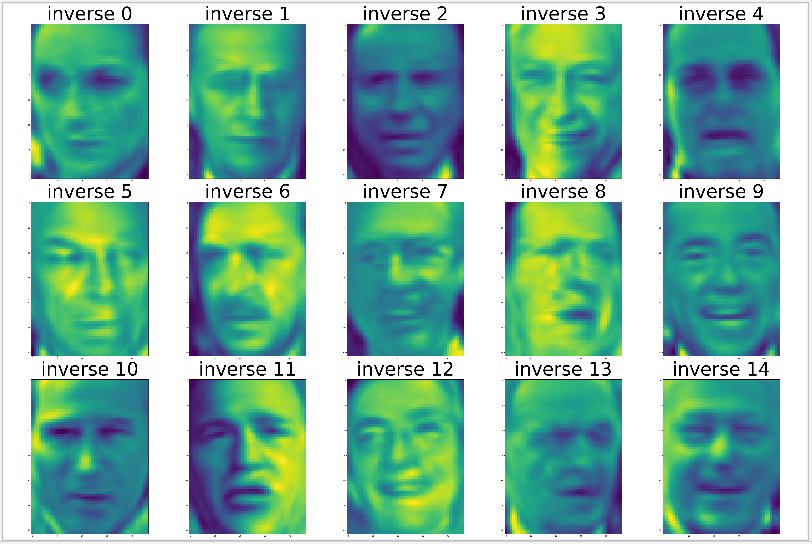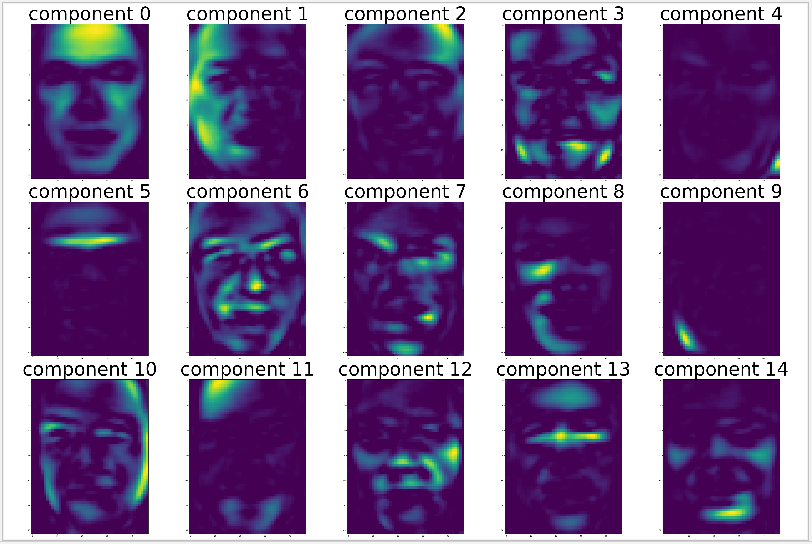2.3.2 还原面部特征

 1 def nmf_test1(n):
2     person=datasets.fetch_lfw_people(min_faces_per_person=20)
3     #建立NMF模型，使用 150 个主要成分
4     nmf=NMF(150,random_state=2)
5     #将数据X转换成降维后的数据
6     componmets=nmf.fit_transform(person.data)
7     #按照所提取特征成分 components[n]倒序排列
8     index=np.argsort(componmets[:,n])[::-1]
9     #提取特征成分后还原数据
10     fix, axes1 = plt.subplots(3, 5, figsize=(60, 40))
11     for ax,index1 in zip(axes1.ravel(),index):
12         a = person.data[index1, :]
13         ax.imshow(person.data[index1, :].reshape(62, 47), cmap='viridis')
14         ax.set_title('original ' + str(index1), fontsize=100)
15     plt.show()
16
17 if __name__ == '__main__':
18     nmf_test1(6)
19     nmf_test1(10)

component 6component 10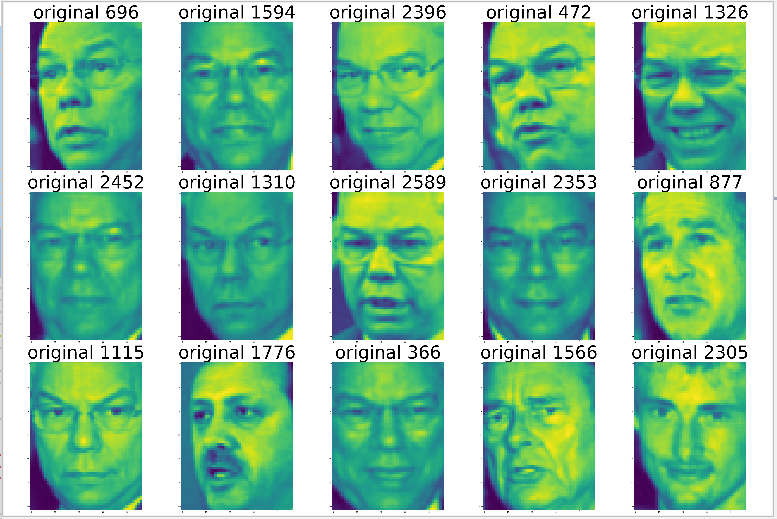PCA、NMF 虽然是比较常用的降维算法，然而对非线性的数据集处理效果不太好，为了弥补这一缺陷 sklearn 提供了另一套方案 —— 流形学习 ML （manifold learning）。流形学习是一种无监督评估器，它试图将一个低维度流形嵌入到一个高维度空间来描述数据集。

3.1 MDS 多维标度法

 1 class MDS(BaseEstimator):
2     @_deprecate_positional_args
3     def __init__(self, n_components=2, *, metric=True, n_init=4,
4                  max_iter=300, verbose=0, eps=1e-3, n_jobs=None,
5                  random_state=None, dissimilarity="euclidean"):
6         self.n_components = n_components
7         self.dissimilarity = dissimilarity
8         self.metric = metric
9         self.n_init = n_init
10         self.max_iter = max_iter
11         self.eps = eps
12         self.verbose = verbose
13         self.n_jobs = n_jobs
14         self.random_state = random_state

• n_components：int, 默认值为2。int 时则是直接指定 NMF 降维后的特征维度数目，此时 n_components是一个大于等于 1 的整数。当使用默认值，即不输入n_components，此时n_components=min(样本数，特征数)。
• dissimilarity：可选值：{'euclidean', 'precomputed'}, 默认值为 'euclidean' 。用于定义模型的非相似性，euclidean：根据点与点之间的欧几里得距离进行计算; precomputed: 根据距离矩阵方式进行试算。
• metric：bool 类型，默认值为True，是否使用距离量度 MDS，False 使用非距离度量 SMACOF
• n_init：int 类型，默认值为 4，初始化 SMACOF 算法方式的运行次数。把应用的最小结果作为运行结果输出。
• max_iter：int 类型，默认值为 300 ，指定了模型优化的最大迭代次数。
• eps：float 类型，默认值为 1-e3，收敛阙值。
• verbose： int类型，默认值为0，代表详细程度。
• n_jobs：CPU 并行数，默认为None，代表1。若设置为 -1 的时候，则用所有 CPU 的内核运行程序。
• random_state：int 类型，默认None，随机数种子，推荐设置一个任意整数，同一个随机值，模型可以复现。

 1 def mds_test():
2     # 测试数据
3     n_points = 1000
4     X, color = datasets._samples_generator.make_s_curve(n_points, random_state=0)
5     # 显示测试数据的3D图
6     ax1 = plt.axes(projection='3d')
7     ax1.scatter3D(X[:, 0], X[:, 1], X[:, 2], c=color)
8     plt.show()
9     # 初始化 MDS 模型
10     mds=MDS(n_components=2,random_state=1)
11     model=mds.fit_transform(X)
12     # 显示 MDS 评估结果
13     ax2=plt.axes()
14     plt.scatter(model[:,0],model[:,1])
15     plt.show()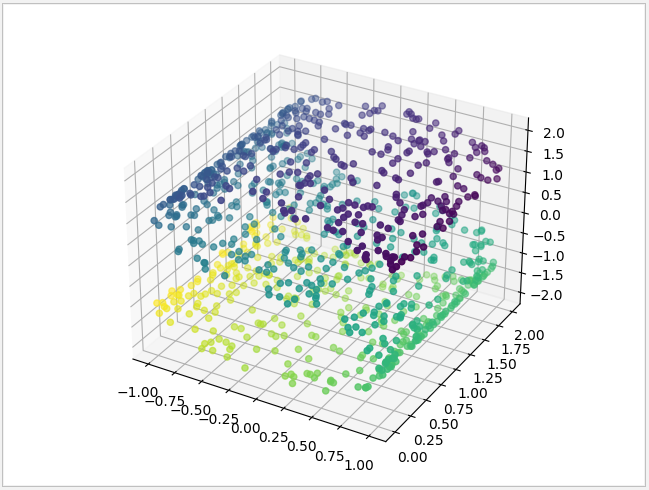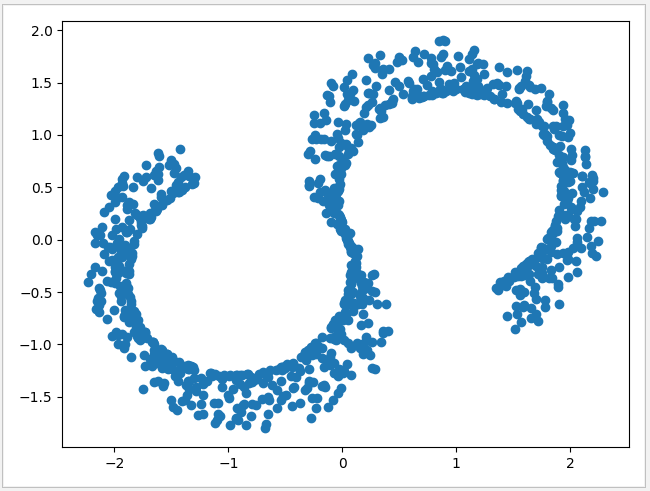3.2  LLE 局部线性嵌入法

 1 class LocallyLinearEmbedding(TransformerMixin,
2                      　　　　_UnstableArchMixin, BaseEstimator):
3 　　@_deprecate_positional_args
4     def __init__(self, *, n_neighbors=5, n_components=2, reg=1E-3,
5                  eigen_solver='auto', tol=1E-6, max_iter=100,
6                  method='standard', hessian_tol=1E-4, modified_tol=1E-12,
7                  neighbors_algorithm='auto', random_state=None, n_jobs=None):
8         ......

• n_neighbors: int, 默认为 5 表示默认邻居的数量。
• n_components：int, 默认值为2。int 时则是直接指定降维后的特征维度数目，此时 n_components是一个大于等于 1 的整数。当使用默认值，即不输入n_components，此时n_components=min(样本数，特征数)。
• reg：float 类型，默认值 1e-3,正则化系数，当 n_neighbors大于n_components时，即近邻数大于降维的维数时，由于我们的样本权重矩阵不是满的，LLE通过正则化来解决这个问题。
• eigen_solver：str 类型， {'auto', 'arpack', 'dense'} 之一, 默认值 'auto' ，特征分解的方法。‘dense’：适合于非稀疏的矩阵分解;  ‘arpack’: 虽然可以适应稀疏和非稀疏的矩阵分解，但在稀疏矩阵分解时会有更好算法速度。
• tol：float 类型，默认值为 1e-6，对当 eigen_solver 为 arpack 时的公差，代表求解方法精度。在 eigen_solver 为 dense 时无效。
• max_iter：int 类型，默认值为 100 ，指定了模型优化的最大迭代次数。
• method：str 类型 {'standard', 'hessian', 'modified', 'ltsa'} 之一, 默认值为 'standard' ，指定 LLE 的具体算法。一般来说  'hessian', 'modified', 'ltsa' 算法在同样的近邻数 n_neighbors 情况下，运行时间会比standard 的 LLE 长，当然降维的效果会稍微好一些。如果你对降维后的数据局部效果很在意，那么可以考虑使用  'hessian', 'modified', 'ltsa' 或者增大n_neighbors，否则标准的 standard 就可以了。需要注意的是使用 modified 要求n_neighbors > n_components，而使用 hessian 要求n_neighbors > n_components * (n_components + 3) / 2
• hessian_tol：float 类型，默认值为 1e-4，当 method 为 hessian 时的公差精度，其他 method 无效
• modified_tol: float 类型，默认值为 1e-12，当 method 为 modified 时的公差精度，其他 method 无效
• neighbors_algorithm：str 类型，{'auto', 'brute', 'kd_tree', 'ball_tree'} 之一, 默认值为 'auto' ，代表 k近邻的实现方法，和KNN算法的使用的搜索方法类似。‘brute’ 对应第一种蛮力实现，‘kd_tree’对应第二种KD树实现，‘ball_tree’对应第三种的球树实现， ‘auto’则会在上面三种算法中做权衡，选择一个拟合最好的最优算法。需要注意的是，如果输入样本特征是稀疏的时候，无论我们选择哪种算法，最后scikit-learn都会去用蛮力实现 ‘brute’。
• n_jobs：CPU 并行数，默认为None，代表1。若设置为 -1 的时候，则用所有 CPU 的内核运行程序。
• random_state：int 类型，默认None，随机数种子，推荐设置一个任意整数，同一个随机值，模型可以复现。

使用与 MDS 相同的例子作为测试，把原来三维空间中的立体数据，经过 LLE 二维评估转换后，投影会转换成可见的 S 形数据。

 1 def lls_test():
2     # 测试数据
3     n_points = 1000
4     X, color = datasets._samples_generator.make_s_curve(n_points, random_state=0)
5     # 显示测试数据的3D图
6     ax1 = plt.axes(projection='3d')
7     ax1.scatter3D(X[:, 0], X[:, 1], X[:, 2], c=color)
8     plt.show()
9     # 初始化 LLE 模型
10     lle=LLE(n_neighbors=299,n_components=2,method='standard',random_state=1)
11     model=lle.fit_transform(X)
12     # 显示 LLE 评估结果
13     ax2=plt.axes()
14     ax2.scatter(model[:,0],model[:,1])
15     plt.show()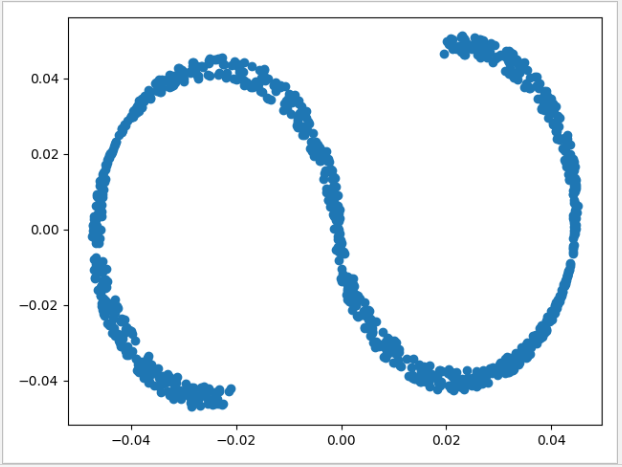3.3 Isomap 保距映射法

Isomap 模型与 LLE 模型相似，数据不会保留所有的距离，而是保留邻节点间的距离，此值可通过参数n_neighbors 设定，默认为5 。 但 Isomap 在现实使用中对高维数据源的学习效果比较差，但有比较好的嵌入效果，一般用于数据集的预处理，可以提供分析数据的线索。

1 class Isomap(TransformerMixin, BaseEstimator):
2     @_deprecate_positional_args
3     def __init__(self, *, n_neighbors=5, n_components=2, eigen_solver='auto',
4                  tol=0, max_iter=None, path_method='auto',
5                  neighbors_algorithm='auto', n_jobs=None, metric='minkowski',
6                  p=2, metric_params=None):
7     ......

• n_neighbors: int, 默认为 5 表示默认邻居的数量。
• n_components：int, 默认值为2。int 时则是直接指定降维后的特征维度数目，此时 n_components是一个大于等于 1 的整数。当使用默认值，即不输入n_components，此时n_components=min(样本数，特征数)。
• eigen_solver：str 类型， {'auto', 'arpack', 'dense'} 之一, 默认值 'auto' ，特征分解的方法。‘dense’：适合于非稀疏的矩阵分解;  ‘arpack’: 虽然可以适应稀疏和非稀疏的矩阵分解，但在稀疏矩阵分解时会有更好算法速度。
• tol：float 类型，默认值为 1e-6，对当 eigen_solver 为 arpack 时的公差，代表求解方法精度。在 eigen_solver 为 dense 时无效。
• max_iter：int 类型，默认值为 None，当 eigen_solver 为 arpack 时，指定了模型优化的最大迭代次数。在 eigen_solver 为 dense 时无效。
• path_method: str 类型，{'auto', 'FW', 'D'} 之一, 默认值为 'auto'。 ‘FW’:使用 Floyd_Warshall 算法 ;  ‘D’: 使用 Dijkstra 算法
• neighbors_algorithm：str 类型，{'auto', 'brute', 'kd_tree', 'ball_tree'} 之一, 默认值为 'auto' ，代表 k近邻的实现方法，和KNN算法的使用的搜索方法类似。‘brute’ 对应第一种蛮力实现，‘kd_tree’对应第二种KD树实现，‘ball_tree’对应第三种的球树实现， ‘auto’则会在上面三种算法中做权衡，选择一个拟合最好的最优算法。需要注意的是，如果输入样本特征是稀疏的时候，无论我们选择哪种算法，最后scikit-learn都会去用蛮力实现 ‘brute’。
• n_jobs：CPU 并行数，默认为None，代表1。若设置为 -1 的时候，则用所有 CPU 的内核运行程序。
• metric： str 类型 或 callable, 默认值为 ‘minkowski’。
• p: int 类型，默认值为2 。
• metric_params: dict类型 , 默认值 为 None，使用 metric 时的参数

 1 def iosmap_test():
2     # 测试数据
3     X, color =make_swiss_roll(n_samples=2000, noise=0.1)
4     # 显示测试数据的3D图
5     ax1 = plt.axes(projection='3d')
6     ax1.scatter3D(X[:, 0], X[:, 1], X[:, 2], c=color)
7     plt.show()
8     # 初始化 Isomap 模型
9     isomap =Isomap(n_neighbors=299,n_components=2)
10     model=isomap.fit_transform(X)
11     # 显示 Isomap 评估结果
12     ax2=plt.axes()
13     ax2.scatter(model[:,0],model[:,1])
14     plt.show()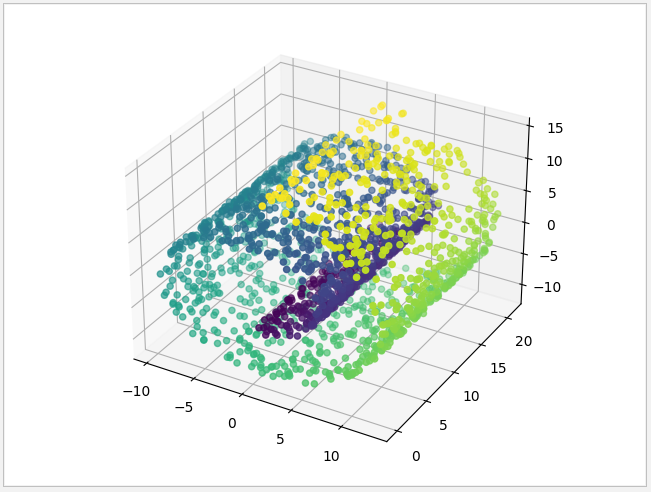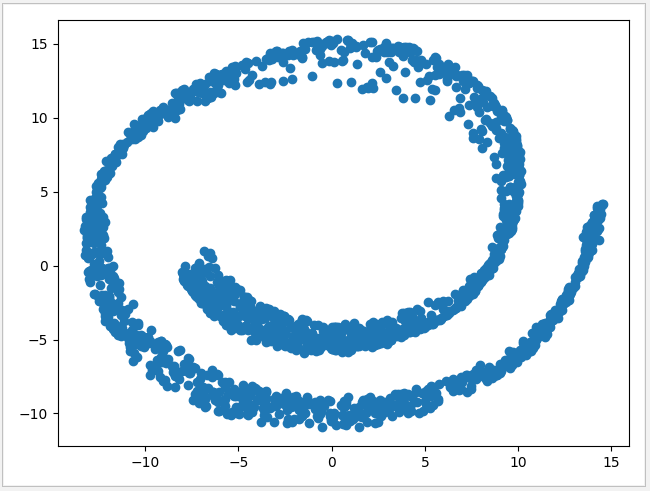3.4 t-SNE 分布邻域嵌入算法

t-SNE 分布邻域嵌入算法的思想是找到数据的一个二维表示方法，尽可能地分析数据点之间的距离，让原始特征中距离较近的点更加近，距离较远的点更加远。通过加大距离差值，更有利于分析特征差异。

1 class TSNE(BaseEstimator):
2     @_deprecate_positional_args
3     def __init__(self, n_components=2, *, perplexity=30.0,
4                  early_exaggeration=12.0, learning_rate=200.0, n_iter=1000,
6                  metric="euclidean", init="random", verbose=0,
7                  random_state=None, method='barnes_hut', angle=0.5,
8                  n_jobs=None, square_distances='legacy'):

• n_components：int, 默认值为2。int 时则是直接指定降维后的特征维度数目，此时 n_components是一个大于等于 1 的整数。当使用默认值，即不输入n_components，此时n_components=min(样本数，特征数)。
• perplexity：float 类型，默认值为 30.0，较大的数据集通常需要更大的perplexity，一般介于5和50之间的值。
• early_exaggeration: float 类型，默认值为12.0 。控制原始空间中的自然簇在嵌入空间中的紧密程度以及它们之间的空间大小。对于较大的值，自然簇之间的空间将在嵌入空间中更大。同样，这个参数的选择不是很关键。如果在初始优化时成本函数增加，则早期夸大因子或学习率可能过高。
• learning_rate：float 类型，默认值为 200.0 表示学习率。学习率太高了，数据可能看起来像一个“球”形与它的最近邻大约相等的距离。如果是学习率太低了，大多数点可能看起来被压缩在一个密集的区域，几乎没有异常值，如果遇到此情形可尝试提高学习率可能会有所帮助。
• n_iter：int 类型，默认值为1000，优化的最大迭代次数，一般应该在 200 以上。
• n_iter_without_progress：int 类型，默认值为 30，控制迭代停止条件。
• metric：str 类型或 callable 默认值为 euclidean，表示计算特征数组中实例之间的距离时使用的度量为欧氏距离的平方。如果度量标准是字符串，则它必须是scipy.spatial.distance.pdist为其度量标准参数所允许的选项之一，或者是成对列出的度量标准.PAIRWISE_DISTANCE_FUNCTIONS。如果度量是“预先计算的”，则X被假定为距离矩阵。或者，如果度量标准是可调用函数，则会在每对实例（行）上调用它，并记录结果值。可调用应该从X中获取两个数组作为输入，并返回一个表示它们之间距离的值。
• init：str 类型，{'random', 'pca'}之一，或 narray 类型数组 (n_samples，n_components)，默认值为 random，嵌入的初始化。PCA 初始化不能与预先计算的距离一起使用，通常比随机初始化更全局稳定。
• verbose：int 类型，默认值为 0，详细度等级。
• random_state: ：int 类型，默认None，随机数种子，推荐设置一个任意整数，同一个随机值，模型可以复现。
• method：str 类型，默认值为 “barnes_hut”，默认情况下，梯度计算算法使用在O（NlogN）时间内运行的 Barnes-Hut 近似值。 method ='exact' 将运行在O（N ^ 2）时间内较慢但精确的算法上。当最近邻的误差需要好于3％时，应该使用精确的算法。但是，确切的方法无法扩展到数百万个示例。
• angle：float 类型，默认值为 0.5 。仅当 method ='barnes_hut' 时才有效，这是Barnes-Hut T-SNE的速度和准确性之间的折衷。 'angle'是从一个点测量的远端节点的角度大小（在中称为theta）。如果此大小低于'角度'，则将其用作其中包含的所有点的汇总节点。该方法对0.2-0.8范围内该参数的变化不太敏感。小于0.2的角度会迅速增加计算时间和角度，因此0.8会快速增加误差。
• n_jobs：int 类型，CPU 并行数，默认为None，代表1。若设置为 -1 的时候，则用所有 CPU 的内核运行程序。
• square_distances：True 或 'legacy‘，是否将距离值平方。’legacy‘ 意味着距离值只有在米制=“欧几里德” 时才平方。True表示所有度量的距离值都是平方的。

 1 def tsne_test():
2     # 输入测试数据
4     # Isomap 模型测试
5     isomap=Isomap(n_neighbors=299,n_components=2)
6     model0=isomap.fit_transform(digits.data)
7     # 转出 Isomap 模型测试结果
8     plt.xlim(model0[:,0].min(),model0[:,0].max()+1)
9     plt.ylim(model0[:,1].min(),model0[:,1].max()+1)
10     for i in range(len(model0)):
11         plt.text(model0[i,0],model0[i,1],str(digits.target[i]),
12                 color='red',fontdict={'weight':'bold','size':9})
13     plt.show()
14     # t-SNE 模型测试
15     tsne=TSNE(random_state=2,learning_rate=800)
16     model1=tsne.fit_transform(digits.data)
17     plt.figure(figsize=(6,6))
18     #输出 t-SNE 测试结果
19     plt.xlim(model1[:,0].min(),model1[:,0].max()+1)
20     plt.ylim(model1[:,1].min(),model1[:,1].max()+1)
21     for i in range(len(model1)):
22         plt.text(model1[i,0],model1[i,1],str(digits.target[i]),
23                 color='red',fontdict={'weight':'bold','size':8})
24     plt.show()`

Isomap 测试结果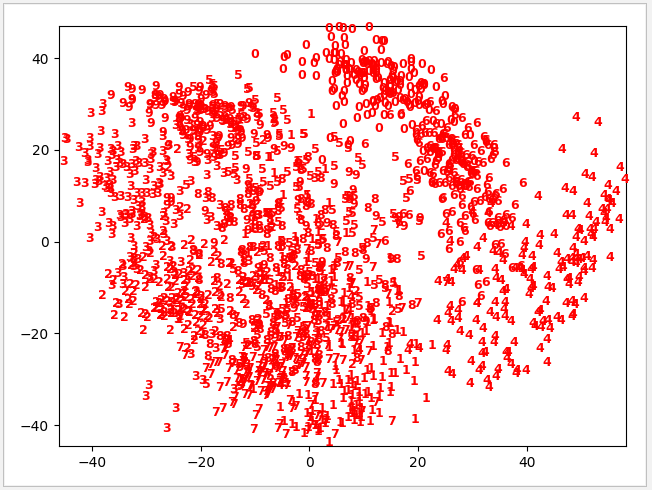t-SNE 测试结果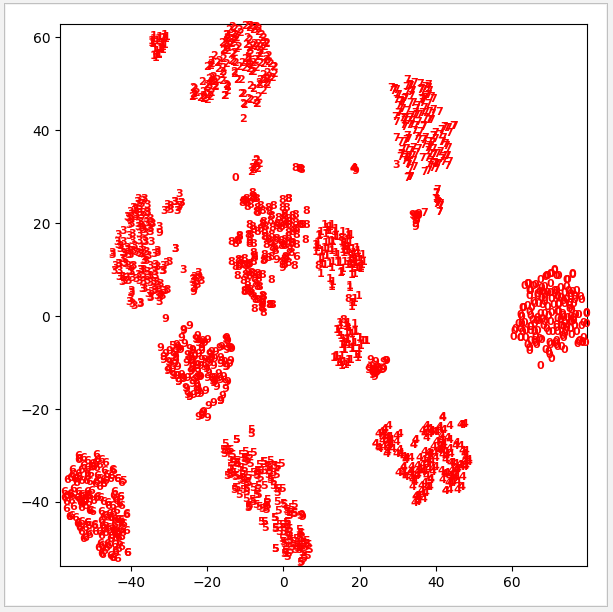AI 人工智能相关文章

Python 机器学习实战 —— 监督学习（上）

Python 机器学习实战 —— 监督学习（下）

Python 机器学习实战 —— 无监督学习（上）

Python 机器学习实战 —— 无监督学习（下）

Tensorflow 2.0 深度学习实战——介绍损失函数、优化器、激活函数、多层感知机的实现原理

https://www.cnblogs.com/leslies2/p/15035784.html

posted on 2021-07-28 17:59  风尘浪子  阅读(581)  评论(2编辑  收藏  举报